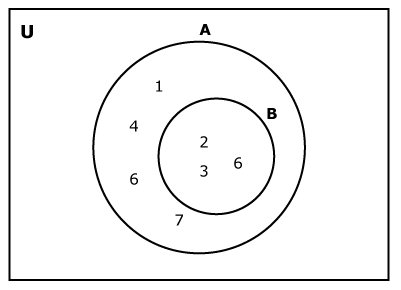# Python Set issuperset()

The issuperset() method returns True if a set has every elements of another set (passed as an argument). If not, it returns False.

Set A is said to be the superset of set B if all elements of B are in A.Here, set A is a superset of set B and B is a subset of set A.

The syntax of issuperset() is:

`A.issuperset(B)`

The following code checks if A is a superset of B.

## Return Value from issuperset()

The issuperset() returns

• True if A is a superset of B
• False if A is not a superset of B

## Example: How issuperset() works?

```A = {1, 2, 3, 4, 5}
B = {1, 2, 3}
C = {1, 2, 3}

# Returns True
print(A.issuperset(B))

# Returns False
print(B.issuperset(A))

# Returns True
print(C.issuperset(B))
```

When you run the program, the output will be:

```True
False
True```

If you need to check if a set is a subset of another set, you can use issubset() in Python.